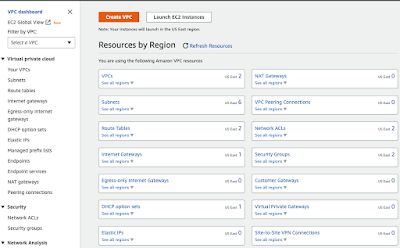### Implement and configure AWS Backup for VMware Cloud on AWS VM workloadsIn our previous post we saw the design of the AWS Backup on VMC. In this post we’re going through the implementation steps As per the design and best practice, we are going to use the ENI for the Backup traffic CREATE A VPC ENDPOINT  TO CREATE AN INTERFACE ENDPOINT FOR AN AWS SERVICE 1. Open the Amazon VPC console at https://console.aws.amazon.com/vpc    2. In the navigation pane, choose Endpoints 3. Choose Create endpoint 4. Name the endpoint   5. For Service category, choose AWS services 6. For Service name, search “ Backup ” and select “ backup-gateway ” service from the dropdown 7. For VPC, select the VPC which we used for SDDC deployment and extension 8. To create an interface endpoint for Amazon S3, you must “uncheck” Additional settings, Enable DNS name. This is because Amazon S3 does not support private DNS for interface VPC endpoints 9. For  Subnets , select one subnet per Availability Zone which we used for SDDC VMC selection  10. For Security group , sel

### Convert an IP Address from Decimal to Binary Form

1. The first, and probably most important step, is to put down this row of values:
 128 64 32 16 8 4 2 1
1. Question: Can I subtract 128 from 154? Answer: YES. So we assign 1 to 128.
 128 64 32 16 8 4 2 1 1
2. Question: Can I subtract 64 from 26? Answer: NO. So we assign 0 to 64.
 128 64 32 16 8 4 2 1 1 0
3. Question: Can I subtract 32 from 26? Answer: NO. So we assign 0 to 32.
 128 64 32 16 8 4 2 1 1 0 0
4. Question: Can I subtract 16 from 26? Answer: YES. So we assign 1 to 16.
 128 64 32 16 8 4 2 1 1 0 0 1
5. That will give us a remainder of 10. (26-16=10). Question: Can I subtract 8 from 10? Answer: YES. So we assign 1 to 8.
 128 64 32 16 8 4 2 1 1 0 0 1 1
6. That will give us a remainder of 2. (10-8=2). Question: Can I subtract 4 from 2? Answer: NO. So we assign 0 to 4.
 128 64 32 16 8 4 2 1 1 0 0 1 1 0
7. Question: can I subtract 2 from 2? Answer: YES. So we assign 1 to 2.
 128 64 32 16 8 4 2 1 1 0 0 1 1 0 1
8. That will give us a remainder of 0. So for the rest of the values in our row, we can assign 0.
 128 64 32 16 8 4 2 1 1 0 0 1 1 0 1 0
So now we know that a decimal number 154 is 10011010 converted to binary form. To double check, we take the values assigned with 1 and add them together: 128+16+8+2=154
3. Our next number in the IP address is: 31. So we start with a question from step 2 again
1. Can I subtract 128 from 31?
 128 64 32 16 8 4 2 1 0
2. Can I subtract 64 from 31?
 128 64 32 16 8 4 2 1 0 0
3. Can I subtract 32 from 31?
 128 64 32 16 8 4 2 1 0 0 0
4. Can I subtract 16 from 31?
 128 64 32 16 8 4 2 1 0 0 0 1
5. Can I subtract 8 from 15 (remember, it’s the remainder)?
 128 64 32 16 8 4 2 1 0 0 0 1 1
6. Can I subtract 4 from 7?
 128 64 32 16 8 4 2 1 0 0 0 1 1 1
7. Can I subtract 2 from 3?
 128 64 32 16 8 4 2 1 0 0 0 1 1 1 1
8. Can I subtract 1 from 1?
 128 64 32 16 8 4 2 1 0 0 0 1 1 1 1 1
So the decimal number 31 is 00011111 converted to binary form. To double check: 16+8+4+2+1=31
4. Next number is 16. I will perform the conversion in one step now.
 128 64 32 16 8 4 2 1 0 0 0 1 0 0 0 0
So the decimal number 16 is 00010000 converted to binary form.
5. Next number is 13.
 128 64 32 16 8 4 2 1 0 0 0 0 1 1 0 1
So the decimal number 13 is 00001101 in binary form. To double check: 8+4+1=13
So the IP address of 154.31.16.13 has its binary form equivalent of:
10011010.00011111.00010000.00001101